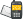It is currently 25 Nov 2020, 19:36### GMAT Club Daily Prep

#### Thank you for using the timer - this advanced tool can estimate your performance and suggest more practice questions. We have subscribed you to Daily Prep Questions via email.

Customized
for You

we will pick new questions that match your level based on your Timer History

Track

every week, we’ll send you an estimated GMAT score based on your performance

Practice
Pays

we will pick new questions that match your level based on your Timer History

#### Not interested in getting valuable practice questions and articles delivered to your email? No problem, unsubscribe here.# What is the volume of the largest cube that can fitQuestion banks Downloads My Bookmarks Reviews Important topics
Author Message
TAGS:GRE InstructorJoined: 10 Apr 2015
Posts: 3907
Followers: 163

Kudos [?]: 4766  , given: 70

What is the volume of the largest cube that can fit [#permalink]
1
KUDOS
Expert's post00:00

Question Stats:43% (01:58) correct56% (00:58) wrongbased on 32 sessions
What is the volume of the largest cube that can fit inside a cylinder with radius 2 and height 3?

A) 9
B) 8√2
C) 16
D) 16√2
E) 27
[Reveal] Spoiler: OA

_________________

Brent Hanneson – Creator of greenlighttestprep.comModeratorJoined: 07 Jan 2018
Posts: 697
Followers: 11

Kudos [?]: 785  , given: 88

Re: What is the volume of the largest cube that can fit [#permalink]
1
KUDOS
Hello Brent can you put an ans to this question.

Posted from my mobile deviceGRE InstructorJoined: 10 Apr 2015
Posts: 3907
Followers: 163

Kudos [?]: 4766  , given: 70

Re: What is the volume of the largest cube that can fit [#permalink]
2
KUDOS
Expert's post
GreenlightTestPrep wrote:
What is the volume of the largest cube that can fit inside a cylinder with radius 2 and height 3?

A) 9
B) 8√2
C) 16
D) 16√2
E) 27

Let's first inscribe the largest possible square inside the circleSince the radius of the cylinder is 2, we know that the DIAMETER = 4Since we have a RIGHT TRIANGLE, we can apply the Pythagorean Theorem to get: x² + x² = 4²
Simplify: 2x² = 16
So, x² = 8, which means x = √8 = 2√2

ASIDE: On test day, you should know the following approximations:
√2 ≈ 1.4
√3 ≈ 1.7
√5 ≈ 2.2

So, we get: x = 2√2 ≈ 2(1.4) ≈ 2.8At this point, we should recognize that, since the height of the cylinder is 3......then the LARGEST CUBE will have dimensions 2√2 by 2√2 by 2√2

Volume = (2√2)(2√2)(2√2)
= 8√8
= 8(2√2)
= 16√2

Cheers,
Brent
_________________

Brent Hanneson – Creator of greenlighttestprep.comModeratorJoined: 07 Jan 2018
Posts: 697
Followers: 11

Kudos [?]: 785  , given: 88

Re: What is the volume of the largest cube that can fit [#permalink]
1
KUDOS
GreenlightTestPrep wrote:
GreenlightTestPrep wrote:
What is the volume of the largest cube that can fit inside a cylinder with radius 2 and height 3?

A) 9
B) 8√2
C) 16
D) 16√2
E) 27

Let's first inscribe the largest possible square inside the circleSince the radius of the cylinder is 2, we know that the DIAMETER = 4Since we have a RIGHT TRIANGLE, we can apply the Pythagorean Theorem to get: x² + x² = 4²
Simplify: 2x² = 16
So, x² = 8, which means x = √8 = 2√2

ASIDE: On test day, you should know the following approximations:
√2 ≈ 1.4
√3 ≈ 1.7
√5 ≈ 2.2

So, we get: x = 2√2 ≈ 2(1.4) ≈ 2.8At this point, we should recognize that, since the height of the cylinder is 3......then the LARGEST CUBE will have dimensions 2√2 by 2√2 by 2√2

Volume = (2√2)(2√2)(2√2)
= 8√8
= 8(2√2)
= 16√2

Cheers,
Brent

Hello Brent, I have a question isn't the volume of a cylinder with r = 2 and height = 3 = $$pi* r^2 * h$$ and in this case $$pi * 2^2 * 3$$ = $$12pi$$ and hence the volume of the cube should be 12pi or closest value less than that and from the option choice E?
_________________

Need Practice? 20 Free GRE Quant Tests available for free with 20 KudosGRE InstructorJoined: 10 Apr 2015
Posts: 3907
Followers: 163

Kudos [?]: 4766  , given: 70

Re: What is the volume of the largest cube that can fit [#permalink]
2
KUDOS
Expert's post
amorphous wrote:

Hello Brent, I have a question isn't the volume of a cylinder with r = 2 and height = 3 = $$pi* r^2 * h$$ and in this case $$pi * 2^2 * 3$$ = $$12pi$$ and hence the volume of the cube should be 12pi or closest value less than that and from the option choice E?

You're right to say that the correct answer choice be LESS THAN 12pi, but it need not be close to 12pi.

For example, we could have a very wide and very short cylinder with radius 1,000 and height 1. The volume of this cylinder would be HUGE (1,000,000pi to be exact).
However, since we need to place a CUBE inside this cylinder, and since all the sides of a cube must be the same length, the largest possible cube that will fit will be a 1x1x1 cube (with a volume of 1)

So, even though 1 is less than 1,000,000pi, it is not close.

Cheers,
Brent
_________________

Brent Hanneson – Creator of greenlighttestprep.comRe: What is the volume of the largest cube that can fit   [#permalink] 20 Jun 2018, 05:07
Display posts from previous: Sort by

# What is the volume of the largest cube that can fitQuestion banks Downloads My Bookmarks Reviews Important topicsPowered by phpBB © phpBB Group Kindly note that the GRE® test is a registered trademark of the Educational Testing Service®, and this site has neither been reviewed nor endorsed by ETS®.